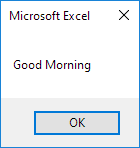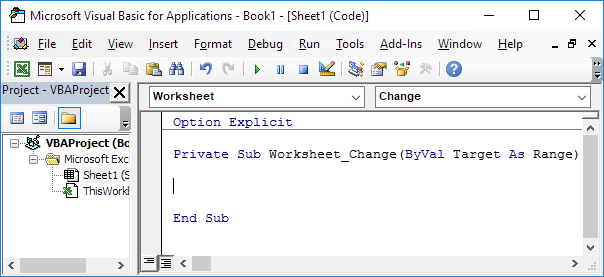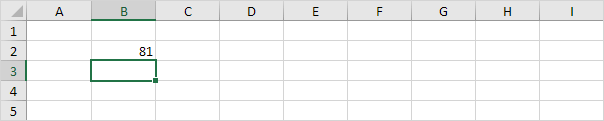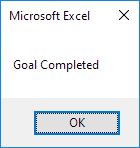# Events

Events are actions performed by users which trigger Excel VBA to execute code.

### Workbook Open Event

Code added to the Workbook Open Event will be executed by Excel VBA when you open the workbook.

1. Open the Visual Basic Editor.

2. Double click on This Workbook in the Project Explorer.

3. Choose Workbook from the left drop-down list. Choose Open from the right drop-down list.4. Add the following code line to the Workbook Open Event:

MsgBox "Good Morning"

5. Save, close and reopen the Excel file.

Result:### Worksheet Change Event

Code added to the Worksheet Change Event will be executed by Excel VBA when you change a cell on a worksheet.

1. Open the Visual Basic Editor.

2. Double click on a sheet (for example Sheet1) in the Project Explorer.

3. Choose Worksheet from the left drop-down list. Choose Change from the right drop-down list.Add the following code lines to the Worksheet Change Event:

4. The Worksheet Change Event listens to all changes on Sheet1. We only want Excel VBA to do something if something changes in cell B2. To achieve this, add the following code lines:

If Target.Address = "\$B\$2" Then

End If

5. We only want Excel VBA to show a MsgBox if the user enters a value greater than 80. To achieve this, add the following code line between If and End If.

If Target.Value > 80 Then MsgBox "Goal Completed"

6. On Sheet1, enter a number greater than 80 into cell B2.Result:Next Chapter: Array   |   Become an Excel Pro: 300 Examples